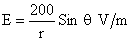Time: 3 Hours                                                                                                     Max. Marks: 100

NOTE: There are 9 Questions in all.

·      Question 1 is compulsory and carries 20 marks. Answer to Q. 1. must be written in the space provided for it in the answer book supplied and nowhere else.

·      Out of the remaining EIGHT Questions answer any FIVE Questions. Each question carries 16 marks.

·      Any required data not explicitly given, may be suitably assumed and stated.

Q.1       Choose the correct or best alternative in the following:                                         (2x10)

a.       Poisson’s equation in CGS Gaussian system is

(A)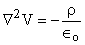(B)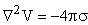(C)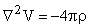(D)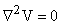b.      The electric potential due to linear quadrapole varies inversely with

(A)    r                                                   (B)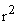(C)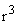(D)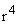c.   In an electromagnetic wave, the phase difference between electric and magnetic field vectors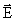and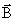is

(A)    zero                                             (B)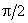(C)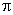(D)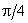d.   If the electrostatic potential is given by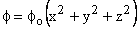where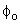is constant, then the charge density giving rise to the above potential would be

(A)    zero                                            (B)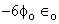(C)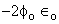(D)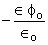e.   Find the amplitude of the electric field in the parallel beam of light of intensity 2.0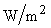.

(A)     28.8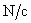(B)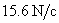(C)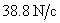(D)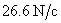f.    The material is described by the following electrical parameters at a frequency of 10 GHz,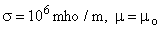and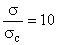, the material at this frequency is considered to be

(A)     a good conductor

(B)     neither a good conductor nor a good dielectric

(C)     a good dielectric

(D)    a good magnetic material

g.   Consider a transmission line of characteristic impedance 50 ohms and the line is terminated at one end by +j50 ohms, the VSWR produced in the transmission line will be

(A)     +1                                               (B)  zero

(C)  infinity                                          (D) -1

h.   A very small thin wire of length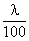has a radiation resistance of

(A)    0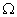(B)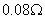(C)  7.9(D)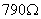i.    Which one of the following conditions will not gurantee a distortionless transmission line

(A)    R = 0 = G

(B)    RC = LG

(C)  very low frequency range (R>>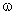L, G >>C)

(D)  very high frequency range (R<<L, G <<C)

j.    The dominant mode of rectangular wave guide is

(A)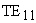(B)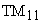(C)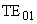(D)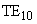Answer any FIVE Questions out of EIGHT Questions.

Each question carries 16 marks.

Q.2     a.   Find the potential inside a uniformly charged sphere considering reference point at infinity.               (10)

b.   A long cylinder carries a charge of density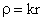, findinside the cylinder.                     (6)

Q.3     a.   Enunciate and give a simple proof for Ampere’s circuital law.                              (9)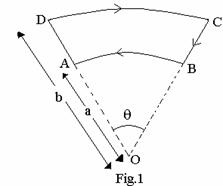b.   A closed current loop having two circular arcs (of radii ‘a’ and ‘b’) and joined by two radial lines as shown in  Fig.1.  Find the magnetic field ‘B’ at the centre O.

(7)

Q.4     a.   What are the speed, direction of propagation and polarisation of an electro-magnetic wave whose electric field components are given as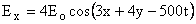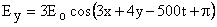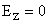(6+2+4)

b.   A plane electromagnetic wave propagating in the x-direction has a wavelength of 5.0 mm.  The electric field is in the y-direction and its maximum magnitude is 30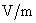.  Write suitable equations for the electric and magnetic fields as a function of x and t. (4)

Q.5     a.   Derive a general expression for reflection coefficient and transmission coefficient forand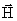fields when an electromagnetic wave is incident parallel (oblique incidence) on the boundary separating two different perfectly dielectric media and also find the expression for normal incidence.                        (8+4)

b.   Find the Laplace equation in spherical polar coordinates for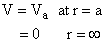(4)

Q.6     a.   Find the condition for distortionless transmission line.  Also calculate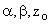for this condition.                                                                    (10)

b.   What length of transmission line should be used at 500 MHz and how should it be terminated for use as a

(i)                  parallel resonant circuit.

(ii)                series resonant circuit.  (6)

Q.7     a.   Find the various field components for a TE wave propagating in a rectangular waveguide.               (8)

b.   In a rectangular waveguide for which a = 1.5 cm, b = 0.8 cm,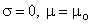and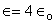and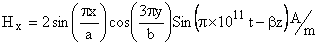Find

(i)                  the mode of operation

(ii)                the cut off frequency

(iii)               phase constant

(iv)              the propagation constant

(v)                the intrinsic wave impedance                                                      (8)

Q.8     a.   What is a linear antenna?  Find out the expression for effective length of linear antenna when

(i)                  the current is distributed along its length and

(ii)                there is sinusoidal distribution of current.

Also find the total power radiated.                                                                      (8)

b.   A small dipole antenna is carrying a uniform r.m.s current of 10 amperes.  Its r.m.s. electric field at a distance ‘r’ metre in a direction making an angle ‘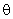’ with the conductor is given by